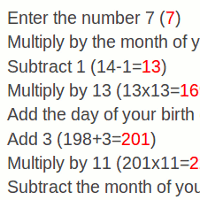# Magical Calculator Birthday Math Trick

### Does a school kid you know want to appear as a maths whiz?

By

Here's a math trick that just might help! Print out this page, and let the kid read carefully the instructions below :

You, the future math whiz, start by giving your friend a calculator. Then, you issue the following sequence of instructions. Make sure your friend presses the equals button (=) after each step!

• Enter the number 7
• Multiply by the month of your birth
• Subtract 1
• Multiply by 13
• Multiply by 11
• Subtract the month of your birth
• Subtract the day of your birth
• Divide by 10
• Divide by 100

And, amazingly, your friend's birthday will appear on the calculator screen! For example, let's suppose your friend was born on February 29. Below, I repeat the sequence of instructions, showing the result at each step in red.

• Enter the number 7 (7)
• Multiply by the month of your birth (7x2=14)
• Subtract 1 (14-1=13)
• Multiply by 13 (13x13=169)
• Multiply by 11 (201x11=2211)
• Subtract the month of your birth (2211-2=2209)
• Subtract the day of your birth (2209-29=2180)
• Divide by 10 (2180/10=218)
• Divide by 100 (229/100 = 2.29)

This will work every time! Try it!

Of course, a real math trick will impress friends by giving you a real aura of genius! To do this, don't let your friend do the last three steps - do them yourself in your head. Dividing by 10 or 100 or adding 11 should beeasy enough for a kid your age to accomplish in your head, right? Or if you're not so sure, practice a bit with the adult who gave you this.

So now, the instructions become :

• Enter the number 7
• Multiply by the month of your birth (Eg, if your birthday was 29 February, you'd multiply by 2)
• Subtract 1
• Multiply by 13
• Add the day of your birth (eg, if your birthday was 29 February, you'd multiply by 29)
• Multiply by 11
• Subtract the month of your birth (eg 2)
• Subtract the day of your birth (eg 29)
• Tell me the result
• (after mentally dividing by 10, adding 11, and dividing by 100) Your birthday is February 29!

If you are in Europe or Australia, you probably want February 29 to look like 29.02, not 2.29. In that case, wherever you see month, write day, and vice-versa. For example :

• Enter the number 7 (7)
• Multiply by the day of your birth (7x29=203)
• Subtract 1 (203-1=202)
• Multiply by 13 (202x13=2626)
• Multiply by 11 (2631x11=28941)
• Subtract the day of your birth (28941-29=28912)
• Subtract the month of your birth (28912-2=28910)
• Tell me the result
Then, mentally, do these steps
• Divide by 10 (28910/10=2891)